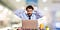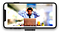Simple low-level Image Processing in Swift with UIKit

Ever thought about creating your own Instagram or Image Filtering application but didn’t exactly know how to approach it? Have some old C++ code, but don’t wanna go through all the wrappers? This guide is meant to show some of the basics of Image Processing all in Swift using only UIKit.

Computers use pixels to display an image. In general, a pixel(smallest controllable element) in an image is composed of 3 or 4 colors of varying intensities:
Red, Green, and Blue (RGB)
or
Cyan, Magenta, Yellow, and Black

The number of distinct colors that can be represented by a pixel depends on the number of bits per pixel (bpp). A 1 bpp image uses 1-bit for each pixel, so each pixel can be either on or off. Each additional bit doubles the number of colors available, so a 2 bpp image can have 4 colors, and a 3 bpp image can have 8 colors.

The basic contrast and brightness adjustments are transformations of the form:

𝑓(𝑥)=𝛼𝑥+𝛽f(x)=αx+β

(with the result rounded to an integer and clamped to the range [0,255][0,255].). Here 𝑥x is a color component value (R,G or B). The slope 𝛼α controls contrast (𝛼>1α>1 means more contrast and 0<𝛼<10<α<1 less contrast). For easier separation of “brightness” and “contrast” modifications, the formula can be written like

𝑓(𝑥)=𝛼(𝑥−128)+128+𝑏f(x)=α(x−128)+128+b

where 𝑏b controls brightness.

Now that we have our basics covered, we can dive into the Swift portion!

Let’s grab our image, then take the cgImage of it:This image is pretty accurate of what I have to deal with on a daily basis!
guard let image = UIImage(named: “image”),
let cgImage = image.cgImage
else { return }

We want to redraw this image with a specific Image Core Graphics Context. So let’s generate some things that we will need for this:

var colorSpace = CGColorSpaceCreateDeviceRGB()var bitmapInfo: UInt32 = CGBitmapInfo.byteOrder32Big.rawValuebitmapInfo |= CGImageAlphaInfo.premultipliedLast.rawValue & CGBitmapInfo.alphaInfoMask.rawValuelet width = Int(image.size.width)
let height = Int(image.size.height)
var bytesPerRow = width * 4
let imageData = UnsafeMutablePointer<Pixel>.allocate(capacity: width * height)

By generating an UnsafeMutablePointer with Pixels (We know from before they are composed of RGB values, as well as an Alpha multiplier), we can manipulate CGContext given information about the size and bitmap (32-bit, big endian format).

guard let imageContext = CGContext(
data: imageData,
width: width,
height: height,
bitsPerComponent: 8,
bytesPerRow: bytesPerRow,
space: colorSpace,
bitmapInfo: bitmapInfo
) else { return nil }

After generating our CGContext struct, we are ready to redraw the image.

imageContext.draw(cgImage, in: CGRect(x: 0, y: 0, width: width, height: height))

Now given that the pointer is generated and updated, we can continue to our interface of the buffer by starting at that pointer.

let pixels = UnsafeMutableBufferPointer<Pixel>(start: imageData, count: width * height)

Given this buffer pointer, we can iterate through each pixel to calculate the total individual amounts of RGB pixels.

for y in 0..<height {
for x in 0..<width {
let index = y * width + x
let pixel = pixels[index]
totalRed += Int(pixel.red)
totalGreen += Int(pixel.green)
totalBlue += Int(pixel.blue)
}
}

Now calculate the averages by dividing by the pixel area.

let avgRed = totalRed / pixelArea
let avgGreen = totalGreen / pixelArea
let avgBlue = totalBlue / pixelArea

Finally, we’re ready to manipulate the pixels!

for y in 0..<height {
for x in 0..<width {
let index = y * width + x
var pixel = pixels[index]
let redDelta = Int(pixel.red) - avgRed
let greenDelta = Int(pixel.green) - avgGreen
let blueDelta = Int(pixel.blue) - avgBlue
pixel.red = UInt8(max(min(255, avgRed + 2 * redDelta), 0))
pixel.blue = UInt8(max(min(255, avgBlue + 2 * blueDelta), 0)) . pixel.green = UInt8(max(min(255, avgGreen + 2 * greenDelta), 0))

pixels[index] = pixel
}
}

The above code can be done any way you want to manipulate contrast. In our case, we simply want to increase the contrast. We can do this by adding a delta with a multiplier of 2 to each of the pixels. Remember the max value is 255, and the min is 0.

Now we can create the CGContext (like we did in the beginning), and use the makeImage() function to generate an image.

guard let newCGImage = context.makeImage() else { return nil }
return UIImage(cgImage: newCGImage)

Let’s see how the output image turned out on the simulator!Now that we increased the contrast, we can see darker colors.

Great! Let’s try the reverse by decreasing the contrast by the delta.

We decreased the contrast so much it ended up creating a photo negative!

Let’s try Grayscale using this forumla:

𝑦=0.3𝑅+0.6𝐺+0.1𝐵

With the use of Unsafe pointers and CGContext from Core Graphics and a little bit of tinkering with RGB values, we can achieve anything with image filters in Swift!!

There are still some ways we can take the basics further. I did not show any histograms of the images on purpose. In the next guide, we will be diving into the use of histograms and manipulating data with complex algorithms to fix the darkness/brightness of hard to see grayscale images.